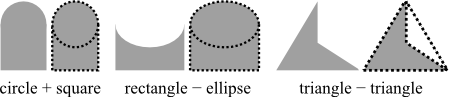# (SOLVED) Complex Shapes

Discipline: Mathematics

Paper Format: APA

Pages: 1 Words: 275

Question

Complex Shapes

Most shapes occurring in the physical world are complex. Complex shapes combine parts or all of simple shapes. These complex shapes include polygons and other shapes that may include parts of circles, squares, triangles, ellipses, and rectangles.To determine the area of a complex shape, use the formulas for simple shapes when possible and either combine or subtract them depending on the composition of the complex shape. Use Heron's formula to calculate the area of a triangle when the lengths of all three sides are known; use Brahmagupta's formula to calculate the area of any quadrilateral given the lengths of the sides and some of their angles.